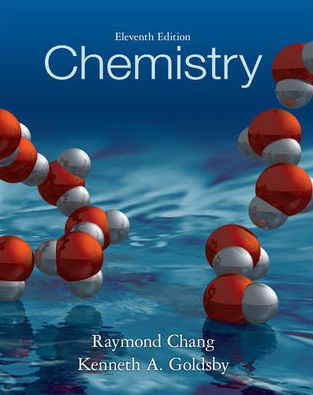### Solution Found!# Molarity Calculations for Various Solutions: CH?OH, CaCl?, C??H?

Chapter 4, Problem 4.66

(choose chapter or problem)

Get Unlimited Answers! Check out our subscriptions
QUESTION:

Calculate the molarity of each of the following solutions:

(a) 6.57 g of methanol $$\left(\mathrm{CH}_{3} \mathrm{OH}\right)$$ in $$1.50 \times 10^{2}$$ mL of solution,

(b) 10.4 g of calcium chloride $$\left(\mathrm{CaCl}_{2}\right)$$ in $$2.20 \times 10^{2}$$ mL of solution,

(c) 7.82 g of naphthalene $$\left(\mathrm{C}_{10} \mathrm{H}_{8}\right)$$ in 85.2 mL of benzene solution.

##### Molarity Calculations for Various Solutions: CH?OH, CaCl?, C??H?Want To Learn More? To watch the entire video and ALL of the videos in the series:

Learn how to calculate the molarity of different solutions containing methanol, calcium chloride, and naphthalene. Essential for chemistry enthusiasts.

QUESTION:

Calculate the molarity of each of the following solutions:

(a) 6.57 g of methanol $$\left(\mathrm{CH}_{3} \mathrm{OH}\right)$$ in $$1.50 \times 10^{2}$$ mL of solution,

(b) 10.4 g of calcium chloride $$\left(\mathrm{CaCl}_{2}\right)$$ in $$2.20 \times 10^{2}$$ mL of solution,

(c) 7.82 g of naphthalene $$\left(\mathrm{C}_{10} \mathrm{H}_{8}\right)$$ in 85.2 mL of benzene solution.

Step 1 of 3

Here we have to calculate the molarity of each of the following solutions

(a) $$6.57 \mathrm{~g} \text { of methanol }\left(\mathrm{CH}_{3} \mathrm{OH}\right) \text { in } 1.50 \times 10^{2} \mathrm{~mL}$$ of solution

It is known that the molarity of a solution can be calculated as follows,

$$\text { Molarity }=\frac{\text { moles of solute }}{\text { Volume of solution }}$$

Here it has been given that,

$$\text { Mass of methanol }=6.57 \mathrm{~g}$$

$$\text { Volume }=1.50 \times 10^{2} \mathrm{~mL}=1.50 \times 10^{1} \mathrm{~L} \simeq 0.150 \mathrm{~L}$$

$$\text { Molecular weight of methanol }=32.042 \mathrm{~g} / \mathrm{mol}$$

In order to calculate the molarity, 1st we have to find out the number of moles,

\begin{aligned} \text { Number of moles of } \mathrm{CH}_{3} \mathrm{OH} & =\frac{\text { Given mass of } \mathrm{C} \mathrm{H}_{3} \mathrm{OH}}{\text { Molecular mass of } \mathrm{C} \mathrm{H}_{3} \mathrm{OH}} \\ & =\frac{6.57 \mathrm{~g}}{32.042 \mathrm{~g} / \mathrm{mol}}=0.205 \mathrm{~mol} \text { of } \mathrm{CH}_{3} \mathrm{OH} \end{aligned}

Now the molarity can be calculated as,

\begin{aligned} \text { Molarity } & =\frac{\text { moles of solute }}{\text { Volume of solution }} \\ & =\frac{0.205 \text { mol of } \mathrm{C} \mathrm{H}_{3} \mathrm{OH}}{0.150 \mathrm{~L}} \\ & =1.37 \mathrm{M} \end{aligned}

Thus the molarity of methanol is found to be 1.37 M